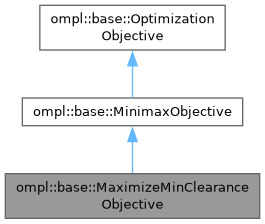ompl::base::MaximizeMinClearanceObjective Class Reference

Objective for attempting to maximize the minimum clearance along a path. More...

#include <ompl/base/objectives/MaximizeMinClearanceObjective.h>

Inheritance diagram for ompl::base::MaximizeMinClearanceObjective:[legend]

## Public Member Functions

MaximizeMinClearanceObjective (const SpaceInformationPtr &si)

Cost stateCost (const State *s) const override
Defined as the clearance of the state s, which is computed using the StateValidityChecker in this objective's SpaceInformation.

bool isCostBetterThan (Cost c1, Cost c2) const override
Since we wish to maximize clearance, and costs are equivalent to path clearance, we return the greater of the two cost values.

Cost identityCost () const override
Returns +infinity, since any cost combined with +infinity under this objective will always return the other cost.

Cost infiniteCost () const override
Returns -infinity, since no path clearance value can be considered worse than this.Public Member Functions inherited from ompl::base::MinimaxObjective
MinimaxObjective (const SpaceInformationPtr &si)

Cost stateCost (const State *s) const override
Returns a cost with a value of 1.

Cost motionCost (const State *s1, const State *s2) const override
Interpolates between s1 and s2 to check for state costs along the motion between the two states. Assumes all costs are worse than identity.

Cost combineCosts (Cost c1, Cost c2) const override
Since we're only concerned about the "worst" state cost in the path, combining two costs just returns the worse of the two.Public Member Functions inherited from ompl::base::OptimizationObjective
OptimizationObjective (const OptimizationObjective &)=delete

OptimizationObjectiveoperator= (const OptimizationObjective &)=delete

OptimizationObjective (SpaceInformationPtr si)
Constructor. The objective must always know the space information it is part of. The cost threshold for objective satisfaction defaults to 0.0.

const std::string & getDescription () const
Get the description of this optimization objective.

virtual bool isSatisfied (Cost c) const
Check if the the given cost c satisfies the specified cost objective, defined as better than the specified threshold.

Cost getCostThreshold () const
Returns the cost threshold currently being checked for objective satisfaction.

void setCostThreshold (Cost c)
Set the cost threshold for objective satisfaction. When a path is found with a cost better than the cost threshold, the objective is considered satisfied.

virtual bool isCostEquivalentTo (Cost c1, Cost c2) const
Compare whether cost c1 and cost c2 are equivalent. By default defined as !isCostBetterThan(c1, c2) && !isCostBetterThan(c2, c1), as if c1 is not better than c2, and c2 is not better than c1, then they are equal.

virtual bool isFinite (Cost cost) const
Returns whether the cost is finite or not.

virtual Cost betterCost (Cost c1, Cost c2) const
Return the minimum cost given c1 and c2. Uses isCostBetterThan.

virtual Cost controlCost (const control::Control *c, unsigned int steps) const
Get the cost that corresponds to the motion created by a control c applied for duration steps. The default implementation uses the identityCost.

virtual Cost initialCost (const State *s) const
Returns a cost value corresponding to starting at a state s. No optimal planners currently support this method. Defaults to returning the objective's identity cost.

virtual Cost terminalCost (const State *s) const
Returns a cost value corresponding to a path ending at a state s. No optimal planners currently support this method. Defaults to returning the objective's identity cost.

virtual bool isSymmetric () const
Check if this objective has a symmetric cost metric, i.e. motionCost(s1, s2) = motionCost(s2, s1). Default implementation returns whether the underlying state space has symmetric interpolation.

virtual Cost averageStateCost (unsigned int numStates) const
Compute the average state cost of this objective by taking a sample of numStates states.

void setCostToGoHeuristic (const CostToGoHeuristic &costToGo)
Set the cost-to-go heuristic function for this objective. The cost-to-go heuristic is a function which returns an admissible estimate of the optimal path cost from a given state to a goal, where "admissible" means that the estimated cost is always less than the true optimal cost.

bool hasCostToGoHeuristic () const
Check if this objective has a cost-to-go heuristic function.

Cost costToGo (const State *state, const Goal *goal) const
Uses a cost-to-go heuristic to calculate an admissible estimate of the optimal cost from a given state to a given goal. If no cost-to-go heuristic has been specified with setCostToGoHeuristic(), this function just returns the identity cost, which is sure to be an admissible heuristic if there are no negative costs.

virtual Cost motionCostHeuristic (const State *s1, const State *s2) const
Defines an admissible estimate on the optimal cost on the motion between states s1 and s2. An admissible estimate always undervalues the true optimal cost of the motion. Used by some planners to speed up planning. The default implementation of this method returns this objective's identity cost, which is sure to be an admissible heuristic if there are no negative costs.

const SpaceInformationPtrgetSpaceInformation () const
Returns this objective's SpaceInformation. Needed for operators in MultiOptimizationObjective.

virtual InformedSamplerPtr allocInformedStateSampler (const ProblemDefinitionPtr &probDefn, unsigned int maxNumberCalls) const
Allocate a heuristic-sampling state generator for this cost function, defaults to a basic rejection sampling scheme when the derived class does not provide a better method.

virtual void print (std::ostream &out) constProtected Attributes inherited from ompl::base::OptimizationObjective
SpaceInformationPtr si_
The space information for this objective.

std::string description_
The description of this optimization objective.

Cost threshold_
The cost threshold used for checking whether this objective has been satisfied during planning.

CostToGoHeuristic costToGoFn_
The function used for returning admissible estimates on the optimal cost of the path between a given state and goal.

## Detailed Description

Objective for attempting to maximize the minimum clearance along a path.

Definition at line 111 of file MaximizeMinClearanceObjective.h.

The documentation for this class was generated from the following files: